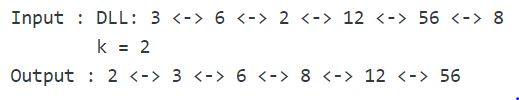# Sort a k sorted doubly linked list

Given a doubly linked list containing n nodes, where each node is at most k away from its target position in the list. The problem is to sort the given doubly linked list.
For example, let us consider k is 2, a node at position 7 in the sorted doubly linked list, can be at positions 5, 6, 7, 8, 9 in the given doubly linked list.

Examples:## Recommended: Please try your approach on {IDE} first, before moving on to the solution.

Naive Approach: Sort the given doubly linked list using insertion sort technique.

Time Complexity: O(nk)
Auxiliary Space: O(1)

Efficient Approach: We can sort the list using the MIN HEAP data structure. The approach has been explained in Sort a nearly sorted (or K sorted) array. We only have to be careful while traversing the input doubly linked list and adjusting the required next and previous links in the final sorted list.

 `// C++ implementation to sort a k sorted doubly ` `// linked list ` `#include ` `using` `namespace` `std; ` ` `  `// a node of the doubly linked list ` `struct` `Node { ` `    ``int` `data; ` `    ``struct` `Node* next; ` `    ``struct` `Node* prev; ` `}; ` ` `  `// 'compare' function used to build up the ` `// priority queue ` `struct` `compare { ` `    ``bool` `operator()(``struct` `Node* p1, ``struct` `Node* p2) ` `    ``{ ` `        ``return` `p1->data > p2->data; ` `    ``} ` `}; ` ` `  `// function to sort a k sorted doubly linked list ` `struct` `Node* sortAKSortedDLL(``struct` `Node* head, ``int` `k) ` `{ ` `    ``// if list is empty ` `    ``if` `(head == NULL) ` `        ``return` `head; ` ` `  `    ``// priority_queue 'pq' implemented as min heap with the ` `    ``// help of 'compare' function ` `    ``priority_queue, compare> pq; ` ` `  `    ``struct` `Node* newHead = NULL, *last; ` ` `  `    ``// Create a Min Heap of first (k+1) elements from ` `    ``// input doubly linked list ` `    ``for` `(``int` `i = 0; head != NULL && i <= k; i++) { ` `        ``// push the node on to 'pq' ` `        ``pq.push(head); ` ` `  `        ``// move to the next node ` `        ``head = head->next; ` `    ``} ` ` `  `    ``// loop till there are elements in 'pq' ` `    ``while` `(!pq.empty()) { ` ` `  `        ``// place root or top of 'pq' at the end of the ` `        ``// result sorted list so far having the first node ` `        ``// pointed to by 'newHead' ` `        ``// and adjust the required links ` `        ``if` `(newHead == NULL) { ` `            ``newHead = pq.top(); ` `            ``newHead->prev = NULL; ` ` `  `            ``// 'last' points to the last node ` `            ``// of the result sorted list so far ` `            ``last = newHead; ` `        ``} ` ` `  `        ``else` `{ ` `            ``last->next = pq.top(); ` `            ``pq.top()->prev = last; ` `            ``last = pq.top(); ` `        ``} ` ` `  `        ``// remove element from 'pq' ` `        ``pq.pop(); ` ` `  `        ``// if there are more nodes left in the input list ` `        ``if` `(head != NULL) { ` `            ``// push the node on to 'pq' ` `            ``pq.push(head); ` ` `  `            ``// move to the next node ` `            ``head = head->next; ` `        ``} ` `    ``} ` ` `  `    ``// making 'next' of last node point to NULL ` `    ``last->next = NULL; ` ` `  `    ``// new head of the required sorted DLL ` `    ``return` `newHead; ` `} ` ` `  `// Function to insert a node at the beginning ` `// of the Doubly Linked List ` `void` `push(``struct` `Node** head_ref, ``int` `new_data) ` `{ ` `    ``// allocate node ` `    ``struct` `Node* new_node =  ` `          ``(``struct` `Node*)``malloc``(``sizeof``(``struct` `Node)); ` ` `  `    ``// put in the data ` `    ``new_node->data = new_data; ` ` `  `    ``// since we are adding at the beginning, ` `    ``// prev is always NULL ` `    ``new_node->prev = NULL; ` ` `  `    ``// link the old list off the new node ` `    ``new_node->next = (*head_ref); ` ` `  `    ``// change prev of head node to new node ` `    ``if` `((*head_ref) != NULL) ` `        ``(*head_ref)->prev = new_node; ` ` `  `    ``// move the head to point to the new node ` `    ``(*head_ref) = new_node; ` `} ` ` `  `// Function to print nodes in a given doubly linked list ` `void` `printList(``struct` `Node* head) ` `{ ` `    ``// if list is empty ` `    ``if` `(head == NULL) ` `        ``cout << ``"Doubly Linked list empty"``; ` ` `  `    ``while` `(head != NULL) { ` `        ``cout << head->data << ``" "``; ` `        ``head = head->next; ` `    ``} ` `} ` ` `  `// Driver program to test above ` `int` `main() ` `{ ` `    ``struct` `Node* head = NULL; ` ` `  `    ``// Create the doubly linked list: ` `    ``// 3<->6<->2<->12<->56<->8 ` `    ``push(&head, 8); ` `    ``push(&head, 56); ` `    ``push(&head, 12); ` `    ``push(&head, 2); ` `    ``push(&head, 6); ` `    ``push(&head, 3); ` ` `  `    ``int` `k = 2; ` ` `  `    ``cout << ``"Original Doubly linked list:n"``; ` `    ``printList(head); ` ` `  `    ``// sort the biotonic DLL ` `    ``head = sortAKSortedDLL(head, k); ` ` `  `    ``cout << ``"\nDoubly linked list after sorting:n"``; ` `    ``printList(head); ` ` `  `    ``return` `0; ` `} `

Output:

```Original Doubly linked list:
3 6 2 12 56 8
2 3 6 8 12 56
```

Time Complexity: O(nLogk)
Auxiliary Space: O(k)

This article is contributed by Ayush Jauhari. If you like GeeksforGeeks and would like to contribute, you can also write an article using contribute.geeksforgeeks.org or mail your article to contribute@geeksforgeeks.org. See your article appearing on the GeeksforGeeks main page and help other Geeks.

Attention reader! Don’t stop learning now. Get hold of all the important DSA concepts with the DSA Self Paced Course at a student-friendly price and become industry ready.

My Personal Notes arrow_drop_up

Improved By : Akanksha_Rai

Article Tags :
Practice Tags :

3

Please write to us at contribute@geeksforgeeks.org to report any issue with the above content.